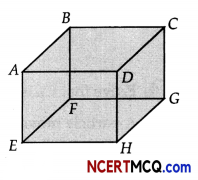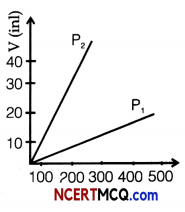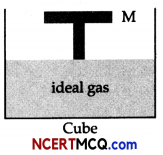Students can access the CBSE Sample Papers for Class 11 Physics with Solutions and marking scheme Term 2 Set 5 will help students in understanding the difficulty level of the exam.

## CBSE Sample Papers for Class 11 Physics Standard Term 2 Set 5 for Practice

Time Allowed: 2 Hours
Maximum Marks: 40

General Instructions:

• There are 12 questions in all. All questions are compulsory.
• This question paper has three sections: Section A, Section B and Section C.
• Section A contains three questions of two marks each, Section B contains eight questions of three marks each, Section C contains one case study-based question of five marks.
• There is no overall choice. However, an internal choice has been provided in one question of two marks and two questions of three marks. You have to attempt only one of the choices in such questions.
• You may use log tables if necessary but use of calculator is not allowed.

Section – A (2 Marks Each)

Question 1.
Why does an iron needle float on clean water but sink when some detergent is added to this water?

Question 2.
Is it possible to increase the temperature of a gas without adding heat to it? If yes, then explain how?
OR
Why does specific heat of gases increase with their atomicity ?

Question 3.
Which is more elastic: steel or rubber? Give reason.

Section – B (3 Marks Each)Question 4.
Prove that CP is greater than CV.

Question 5.
A particle moving in a straight line has velocity υ given by υ2 = α – βy2 , where a and β are constant and y is its distance from a fixed point in the line. Show that the motion of the particle is S.H.M. Find its time period and amplitude.

Question 6.
Derive Charles’s law on the basis of kinetic theory of gases.

Question 7.
(i) Are all periodic functions simple harmonic motion? Give reason for your answer.
(ii) A simple pendulum performs S.H.M. about x = 0 with an amplitude a and time period T. What is the speed of the pendulum at x = a/21Question 8.
Derive an expression for work done in isothermal process.
OR
A hydraulic automobile lift is designed to lift cars with a maximum mass of 3000 kg. The area of cross-section of the piston carrying the load is 425 cm2. What maximum pressure would the smaller piston have to bear?

Question 9.
What is the value of elasticity for
(a) Young’s modulus
(b) Bulk modulus

Question 10.
Derive an expression for fundamental frequency and various overtones of a closed pipe open at one end.

Question 11.
A set of 24 tunning forks is arranged in order of decreasing frequency. Each fork gives 4 beats with succeeding one. The first fork is octave of the last. Calculate the frequency of the first and 12th fork.
OR
Giving reasons for your selection, select pairs out of the following four waves in a medium which will give rise to (i) beats, (ii) destructive interference, (iii) stationary waves.
1. ξ1 = Acos 2π(υ1t + $$\frac{x}{\lambda_{1}}$$)
2. ξ2 = Acos 2π(υ1t + $$\frac{x}{\lambda_{1}}$$ + π)
3. ξ3 = Acos 2π(υ1t + $$\frac{x}{\lambda_{2}}$$)
4. ξ4 = Acos 2π(υ1t + $$\frac{x}{\lambda_{2}}$$)Section – C (5 Marks)

Question 12.
CASE STUDY: PROPERTIES OF AN IDEAL GAS
A cubic vessel ABCDEFGH (with faces horizontal + vertical) contains an ideal gas at NTE The vessel
is being carried by a rocket which is moving up at a speed of 500 ms.
(i) The pressure of the gas inside the vessel as observed by us on the ground
(a) remains the same because 500 ms-1 is very much smaller than vrms of the gas.
(b) remains the same because motion of the vessel does not effect the relative motion of the gas
molecules and the walls.
(c) will increase by a factor equal to(υ2rms + (500)2)/υ2rms where υrms was the original mean square velocity of the gas
(d) will be different on the top and bottom wall of the vessel.

(ii) If the face of cube (EFGH) is made up of a material any gas molecule incident on it. At any given time,(a) pressure on EFGH would be zero.
(b) pressure on all the faces will the equal.
(c) pressure of EFGH =2(Pressure on ABCD)
(d) pressure of EFGH = 1/2 (Pressure on ABCD)

(iii) Gas laws are applicable for ideal gas. Boyle’s law is applicable for an
(b) isothermal process.
(c) isobaric process
(d) isochoric process.

(iv) Volume versus temperature graphs for a given mass of an ideal gas are shown at two different values of constant pressure. What can be inferred about relation between P1 and P2?(a) P1 > P2
(b) P1 = P2
(c) P1 < 2
(d) data is insufficient(v) If the top surface of cube is replaced with frictionless movable piston of mass M, then as temperature is increased,(a) both P and V of the gas will change.
(b) only P will increase according to Charles’s law.
(c) V will change but not P
(d) P will change but not V.